Next: Managing the bisection and Up: Mathematical background Previous: Mathematical background   Contents

Principle

Let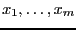be the set of unknowns and let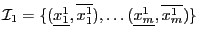be the set of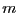intervals in which you are searching the solutions of theequations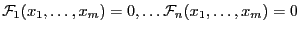(for the sake of simplicity we don't consider inequalities but the extension to inequalities is straightforward).

We will denote by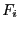the interval value of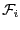when this function is evaluated for the box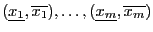of the unknowns while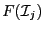will denote the-dimensional interval vector constituted of thewhen the unknowns have the interval value defined by the set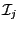.

The algorithm will use a list of boxes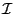whose maximal size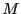is an input of the program. This list is initialized with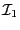. The number ofcurrently in the list is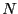and therefore at the start of the program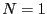. The algorithm will also use an accuracy on the variable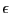and on the functions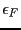. The norm of ais defined as: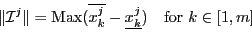The norm of the interval vectoris defined as: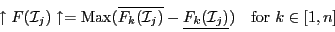The algorithm uses an index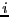and the result is a set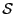of interval vector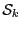for the unknowns whose size is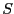. We assume that there is no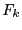with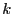in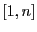such that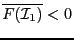or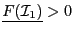, otherwise the equations have no solution in. Two lists of interval vectors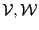whose size is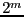will also be used. The algorithm is initialized with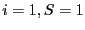and proceed along the following steps:
1. if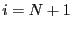return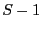andand exit
2. bisect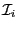which producenew interval vectors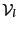and set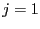3. for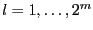1. evaluate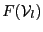2. if it existwithinsuch that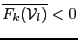or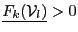, then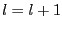and go to step 3
3. if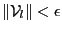or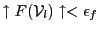, then storein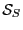, incrementand go to step 3
4. storein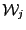, increment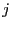and go to step 3
4. ifincrementand go to step 1
5. if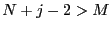return a failure code as there is no space available to store the new intervals
6. if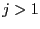store one of the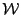in, the other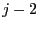at the end of, starting at position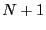. Addtoand go to step 1
Basically the algorithm just bisect the box until either their width is lower thanor the width of the interval function is lower than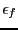(provided that there is enough space in the list to store the intervals). Then if all the intervals functions contain 0 we get a new solution, if one of them does not contain 0 there is no solution of the equations within the current box. A special case occurs when all the components of the box are reduced to a point, in which case a solution is obtained if the absolute value of the interval evaluation of the function is lower than.

Now three problems have to be dealt with:

1. how to choose thewhich will be put in place of theand in which order to store the otherat the end of the list?
2. can we improve the management of the bisection process in order to conclude the algorithm with a limited number?
3. how do we distinguish distinct solutions ?
The first two problems will be addressed in the next section.Next: Managing the bisection and Up: Mathematical background Previous: Mathematical background   Contents
Jean-Pierre Merlet 2012-12-20